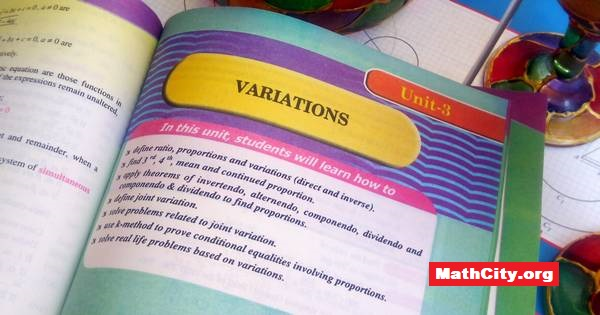# Unit 03: VariationsOn this page the solutions and things related to Unit 03: Variations of Mathematics 10 (Science Group) (Matric) are given. These notes are send by Ms. Maryam Jabeen, we are very thankful to her for sending these notes.

In this unit students will learn how to

• define ratio, proportions and variations (direct and inverse).
• find 3rd, 4th, mean and continued proportion.
• apply theorems of invertendo, alternendo, componendo, dividendo and compnendo and dividendo to find proportions
• define joint variation.
• solve problems related to joint variation.
• use k-method to prove conditional equalities involving proportions.
• solve real life problems based on variations.
• matric/10th_science/unit03